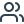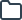CFSD at TU München | Flashcards & Summaries

### Select your language

Suggested languages for you:# Lernmaterialien für CFSD an der TU München

Greife auf kostenlose Karteikarten, Zusammenfassungen, Übungsaufgaben und Altklausuren für deinen CFSD Kurs an der TU München zu.

TESTE DEIN WISSEN

Parabolic PDE

Lösung anzeigen
TESTE DEIN WISSEN
• diffusion phenomena, i.e. the time-dependent solution of an elliptic problem
• Heat conduction
Lösung ausblenden
TESTE DEIN WISSEN

Elliptic partial differential equations (PDE)

Lösung anzeigen
TESTE DEIN WISSEN
• typically model steady-state or equilibrium phenomena
•  Typical equation is the Poisson equation
Lösung ausblenden
TESTE DEIN WISSEN

Typical ill-posed problem

Lösung anzeigen
TESTE DEIN WISSEN

inverse heat conduction

Lösung ausblenden
TESTE DEIN WISSEN

Numerical solution of a well-posed problem can be look like ill-posed if..?

Lösung anzeigen
TESTE DEIN WISSEN

due to numerical instability

Lösung ausblenden
TESTE DEIN WISSEN

conservative

Lösung anzeigen
TESTE DEIN WISSEN

mimics a global conservation law

Lösung ausblenden
TESTE DEIN WISSEN

Loss of theoretical advantages of FEM in CFD due to...

Lösung anzeigen
TESTE DEIN WISSEN

Comparably complex nonlinearities occur in the compressible Euler equations and in the Navier-Stokes equations

Lösung ausblenden
TESTE DEIN WISSEN

dispersion relation

Lösung anzeigen
TESTE DEIN WISSEN

The modified phase velocity is a function of the wavenumber itself

This phenomenon is called dispersion relation of the discretization scheme.

Lösung ausblenden
TESTE DEIN WISSEN

Lax-Equivalence Theorem

Lösung anzeigen
TESTE DEIN WISSEN

Given a well-posed initial-value problem for a linear scalar PDE and a linear discretization scheme of the form (VI.3) that is consistent, LR-stability is necessary and sufficient for convergence.

Lösung ausblenden
TESTE DEIN WISSEN

Numerical schemes need to pass tests and then validated for more general cases under smoothness assumption. WHat are the numerical test equations and testing concepts?

Lösung anzeigen
TESTE DEIN WISSEN

Our numerical test equations are:

o Linear advection equation

o Burgers equation

o 1D Euler equation

Testing concepts of:

o Stability

o Consistency

o Convergence

Lösung ausblenden
TESTE DEIN WISSEN

Concept of linear stability fails for

Lösung anzeigen
TESTE DEIN WISSEN
• Gibbs oscillations caused by Discontinuities
• Regions of sharp solution gradients for which similar reasoning applies
• Compressible flows can develop shocks
Lösung ausblenden
TESTE DEIN WISSEN

Typical well-posed problem

Lösung anzeigen
TESTE DEIN WISSEN

heat conduction

Lösung ausblenden
TESTE DEIN WISSEN

Any system of differential equations describes a well-posed problem for the given initial and boundary conditions given that

Lösung anzeigen
TESTE DEIN WISSEN

• a solution exists,

• the solution is unique,

• the solution depends continuously on the data (initial and boundary conditions)

Lösung ausblenden•522966 Karteikarten
•11317 Studierende
•499 Lernmaterialien

## Beispielhafte Karteikarten für deinen CFSD Kurs an der TU München - von Kommilitonen auf StudySmarter erstellt!

Q:

Parabolic PDE

A:
• diffusion phenomena, i.e. the time-dependent solution of an elliptic problem
• Heat conduction
Q:

Elliptic partial differential equations (PDE)

A:
• typically model steady-state or equilibrium phenomena
•  Typical equation is the Poisson equation
Q:

Typical ill-posed problem

A:

inverse heat conduction

Q:

Numerical solution of a well-posed problem can be look like ill-posed if..?

A:

due to numerical instability

Q:

conservative

A:

mimics a global conservation law

Q:

Loss of theoretical advantages of FEM in CFD due to...

A:

Comparably complex nonlinearities occur in the compressible Euler equations and in the Navier-Stokes equations

Q:

dispersion relation

A:

The modified phase velocity is a function of the wavenumber itself

This phenomenon is called dispersion relation of the discretization scheme.

Q:

Lax-Equivalence Theorem

A:

Given a well-posed initial-value problem for a linear scalar PDE and a linear discretization scheme of the form (VI.3) that is consistent, LR-stability is necessary and sufficient for convergence.

Q:

Numerical schemes need to pass tests and then validated for more general cases under smoothness assumption. WHat are the numerical test equations and testing concepts?

A:

Our numerical test equations are:

o Linear advection equation

o Burgers equation

o 1D Euler equation

Testing concepts of:

o Stability

o Consistency

o Convergence

Q:

Concept of linear stability fails for

A:
• Gibbs oscillations caused by Discontinuities
• Regions of sharp solution gradients for which similar reasoning applies
• Compressible flows can develop shocks
Q:

Typical well-posed problem

A:

heat conduction

Q:

Any system of differential equations describes a well-posed problem for the given initial and boundary conditions given that

A:

• a solution exists,

• the solution is unique,

• the solution depends continuously on the data (initial and boundary conditions)### Erstelle und finde Lernmaterialien auf StudySmarter.

Greife kostenlos auf tausende geteilte Karteikarten, Zusammenfassungen, Altklausuren und mehr zu.

## Das sind die beliebtesten StudySmarter Kurse für deinen Studiengang CFSD an der TU München

Für deinen Studiengang CFSD an der TU München gibt es bereits viele Kurse, die von deinen Kommilitonen auf StudySmarter erstellt wurden. Karteikarten, Zusammenfassungen, Altklausuren, Übungsaufgaben und mehr warten auf dich!

## Das sind die beliebtesten CFSD Kurse im gesamten StudySmarter Universum

##### cf

Universität zu Köln

York University

Hochschule Trier

##### CFD

Hochschule Karlsruhe

## Die all-in-one Lernapp für Studierende

##### Greife auf Millionen geteilter Lernmaterialien der StudySmarter Community zu##### Erstelle Karteikarten und Zusammenfassungen mit den StudySmarter Tools